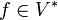# Contragredient representation

## Definition

### Conceptual definition

Suppose$\rho:G \to GL(V)$ is a linear representation of a group$G$ on a vector space$V$ over a field$K$. The contragredient representation of$\rho$, sometimes denoted$\rho^*$, is a linear representation$G \to GL(V^*)$ on the dual space$V^*$ (i.e., the space of linear functionals on$V$) as follows. For$f \in V^*$, i.e.,$f:V \to K$ linear, we define$\rho^*(g)(f)$ as the element of$V^*$ given by$v \mapsto f(g^{-1}(v))$.

### Definition in matrix terms

Suppose$\rho:G \to GL(n,K)$ is a finite-dimensional linear representation of a group$G$ over a field$K$. The contragredient representation$\rho^*: G \to GL(n,K)$ is defined as the composite of the transpose-inverse map with$\rho$, i.e.:$\rho^*(g) = ((\rho(g))^T)^{-1}$

### In the case of a field of characteristic zero closed under complex conjugation

Suppose$K$ is a subfield of the complex numbers that is closed under complex conjugation. Then, the contragredient representation to any representation over$K$ of a finite group$G$ is equivalent to the linear representation obtained by composing complex conjugation with$\rho$.